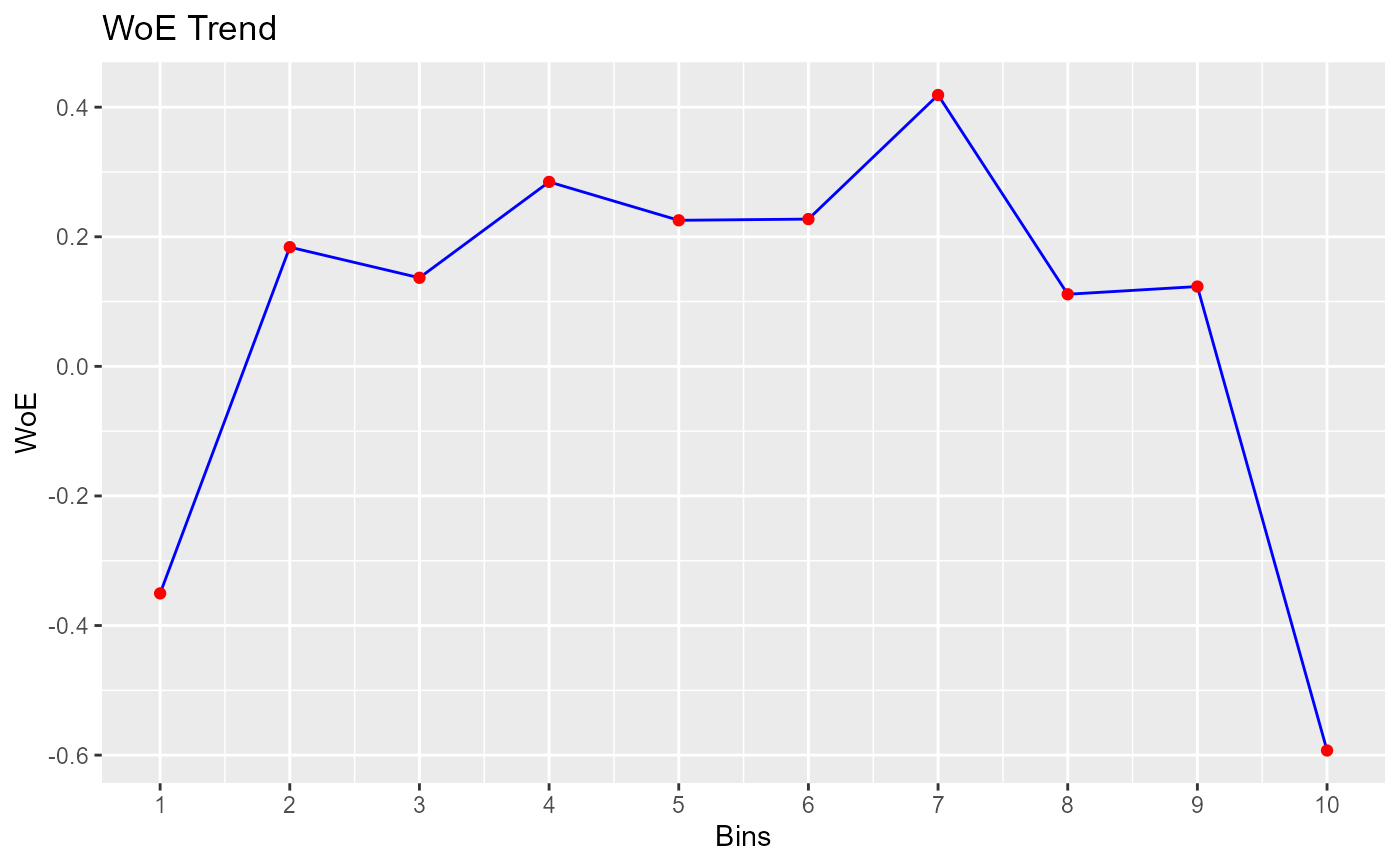Bin continuous data using winsorized method.

## Usage

rbin_winsorize(
data = NULL,
response = NULL,
predictor = NULL,
bins = 10,
include_na = TRUE,
winsor_rate = 0.05,
min_val = NULL,
max_val = NULL,
type = 7,
remove_na = TRUE
)

# S3 method for rbin_winsorize
plot(x, print_plot = TRUE, ...)

## Arguments

data

A data.frame or tibble.

response

Response variable.

predictor

Predictor variable.

bins

Number of bins.

include_na

logical; if TRUE, a separate bin is created for missing values.

winsor_rate

A value from 0.0 to 0.5.

min_val

the low border, all values being lower than this will be replaced by this value. The default is set to the 5 percent quantile of predictor.

max_val

the high border, all values being larger than this will be replaced by this value. The default is set to the 95 percent quantile of predictor.

type

an integer between 1 and 9 selecting one of the nine quantile algorithms detailed in quantile() to be used.

remove_na

logical; if TRUE NAs will removed while calculating quantiles

x

An object of class rbin_winsorize.

print_plot

logical; if TRUE, prints the plot else returns a plot object.

...

further arguments passed to or from other methods.

## Value

A tibble.

## Examples

bins <- rbin_winsorize(mbank, y, age, 10, winsor_rate = 0.05)
bins
#> Binning Summary
#> ------------------------------
#> Method               Winsorize
#> Response             y
#> Predictor            age
#> Bins                 10
#> Count                4521
#> Goods                517
#> Entropy              0.51
#> Information Value    0.1
#>
#>
#>    cut_point bin_count good bad        woe           iv   entropy
#> 1     < 30.2       723  112 611 -0.3504082 0.0224390979 0.6219926
#> 2     < 33.4       567   55 512  0.1839852 0.0039536848 0.4594187
#> 3     < 36.6       573   58 515  0.1367176 0.0022470488 0.4728562
#> 4     < 39.8       497   44 453  0.2846962 0.0079801719 0.4315480
#> 5       < 43       396   37 359  0.2253982 0.0040782670 0.4478305
#> 6     < 46.2       461   43 418  0.2272751 0.0048235624 0.4473095
#> 7     < 49.4       281   22 259  0.4187793 0.0092684760 0.3961315
#> 8     < 52.6       309   32 277  0.1112753 0.0008106706 0.4801796
#> 9     < 55.8       244   25 219  0.1231896 0.0007809490 0.4767424
#> 10   >= 55.8       470   89 381 -0.5928433 0.0456442813 0.7001343

# plot
plot(bins)